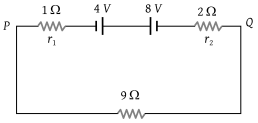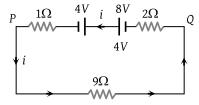NEET Questions Solved

Two batteries of e.m.f. 4V and 8 V with internal resistances 1 Ω and 2 Ω are connected in a circuit with a resistance of 9 Ω as shown in figure. The current and potential difference between the points P and Q are(1)

(2)

(3)

(4) $\frac{1}{2}A\text{\hspace{0.17em}\hspace{0.17em}and\hspace{0.17em}\hspace{0.17em}}12V$

(1) Applying Kirchoff's voltage law in the given loop.$-2i+8-4-1×i-9i=0$$i=\frac{1}{3}A$

Potential difference across PQ $=\frac{1}{3}×9=3V$

Difficulty Level:

• 62%
• 30%
• 3%
• 6%